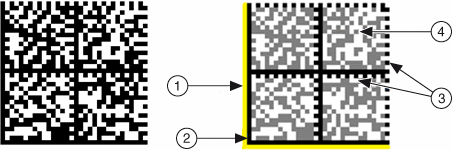# Data Matrix Concepts

NI Vision 2015 Concepts Help

Edition Date: June 2015

Part Number: 372916T-01

»View Product InfoDownload Help (Windows Only)

A Data Matrix code is a matrix built on a square or rectangular grid with a finder pattern around the perimeter of the matrix. Each cell of the matrix contains a single data cell. The cells can be either square or circular.

Locating and decoding Data Matrix codes requires a minimum cell size of 2.5 pixels. Locating and decoding Data Matrix codes also requires a quiet zone of at least one cell width around the perimeter of the code. However, a larger quiet zone increases the likelihood of successful location. Each symbol character value is encoded in a series of data cells called a code word.

Data Matrix codes use one of two error checking and correction (ECC) schemes. Data Matrix codes that use the ECC schemes 000 to 140 are based on the original specification. These codes use a convolution error correction scheme and use a less efficient data packing mechanism that often requires only encoding characters from a particular portion of the ASCII character set. Data Matrix codes that use the ECC 200 scheme use a Reed-Solomon error correction algorithm and a more efficient data packing mechanism. The ECC 200 scheme also allows for the generation of multiple connected matrices, which enables the encoding of larger data sets.

The following figure shows an example of a Data Matrix code:1. Quiet Zone
2. Finder Pattern
3. Clock Pattern
4. Data Cell